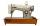# Passenger boat

Two-fifths of the passengers in the passenger boat were boys. 1/3 of them were girls and the rest were adult. If there were 60 passengers in the boat, how many more boys than adult were there?

Correct result:

x =  8

#### Solution:We would be very happy if you find an error in the example, spelling mistakes, or inaccuracies, and please send it to us. We thank you!Tips to related online calculators
Need help calculate sum, simplify or multiply fractions? Try our fraction calculator.

## Next similar math problems:

• TeacherTeacher Rem bought 360 pieces of cupcakes for the outreach program of their school. 5/9 of the cupcakes were chocolate flavor and 1/4 wete pandan flavor and the rest were a vanilla flavor. How much more pandan flavor cupcakes than vanilla flavor?
• Fraction and a decimalWrite as a fraction and a decimal. One and two plus three and five hundredths
• Write 2Write 791 thousandths as fraction in expanded form.
• Fraction to decimalWrite the fraction 3/22 as a decimal.
• ZdeněkZdeněk picked up 15 l of water from a 100-liter full-water barrel. Write a fraction of what part of Zdeněk's water he picked.
• Pizza 4Marcus ate half pizza on monday night. He than ate one third of the remaining pizza on Tuesday. Which of the following expressions show how much pizza marcus ate in total?
• Mixed2improperWrite the mixed number as an improper fraction. 166 2/3
• Lengths of the poolMiguel swam 6 lengths of the pool. Mat swam 3 times as far as Miguel. Lionel swam 1/3 as far as Miguel. How many lengths did mat swim?
• Cupcakes 2Susi has 25 cupcakes. She gives 4/5. How much does she have left?
• WithdrawalIf I withdrew 2/5 of my total savings and spent 7/10 of that amount. What fraction do I have in left in my savings?
• Evaluate expressionCalculate the value of the expression z/3 - 2 z/9 + 1/6, for z = 2
• In fractionsAn ant climbs 2/5 of the pole on the first hour and climbs 1/4 of the pole on the next hour. What part of the pole does the ant climb in two hours?
• Unknown number 6Determine the unknown number, which is by 1.5 greater than its fourth.
• Report 2A School reports students to teacher ratio of 6:1. If there are 45 teachers in the School, how many students are there?
• Sum of fractionsWhat is the sum of 2/3+3/5?
• TailorTailor bought 2 3/4 meters of textile and paid 638 CZK. Determine the price per 1 m of the textile.
• EquationFind x: x + 1/2 = 1/3